#Compound Fractions: ExampleRule 27:
A compound fraction is sometimes called a mixed number.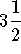,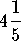, and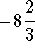are examples of compound fractions.Example:
Convert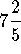to a simple fraction.can be written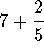. Write 7 as the fraction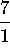.

Nowcan be written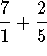.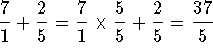.

### If you would like to review another example, click on Example.S.O.S MATHematics home page